# IBM HR Analytics on Employee Attrition & Performance using Random Forest Classifier

• Last Updated : 17 Aug, 2020

Attrition is a problem that impacts all businesses, irrespective of geography, industry and size of the company. It is a major problem to an organization, and predicting turnover is at the forefront of the needs of Human Resources (HR) in many organizations. Organizations face huge costs resulting from employee turnover. With advances in machine learning and data science, it’s possible to predict the employee attrition, and we will predict using Random Forest Classifier algorithm.

Dataset: The dataset that is published by the Human Resource department of IBM is made available at Kaggle.

Code: Implementation of Random Forest Classifier algorithm for classification.

## Python3

 `# performing linear algebra``import` `numpy as np`` ` `# data processing``import` `pandas as pd`` ` `# visualisation``import` `matplotlib.pyplot as plt``import` `seaborn as sns ``%` `matplotlib inline`

Code: Importing the dataset

## Python3

 `dataset ``=` `pd.read_csv(``"WA_Fn-UseC_-HR-Employee-Attrition.csv"``)``print` `(dataset.head)`

Output :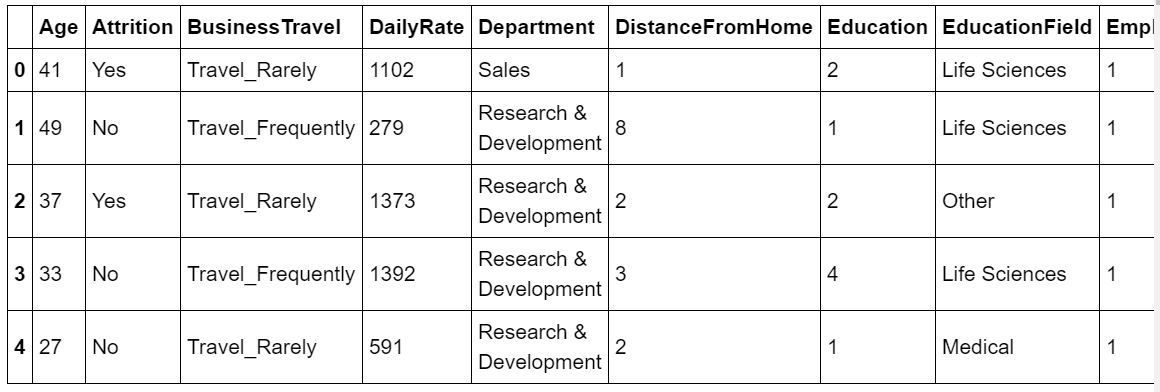Code: Information about the dataset

## Python3

 `dataset.info()`

Output :

```RangeIndex: 1470 entries, 0 to 1469
Data columns (total 35 columns):
Age                         1470 non-null int64
Attrition                   1470 non-null object
BusinessTravel              1470 non-null object
DailyRate                   1470 non-null int64
Department                  1470 non-null object
DistanceFromHome            1470 non-null int64
Education                   1470 non-null int64
EducationField              1470 non-null object
EmployeeCount               1470 non-null int64
EmployeeNumber              1470 non-null int64
EnvironmentSatisfaction     1470 non-null int64
Gender                      1470 non-null object
HourlyRate                  1470 non-null int64
JobInvolvement              1470 non-null int64
JobLevel                    1470 non-null int64
JobRole                     1470 non-null object
JobSatisfaction             1470 non-null int64
MaritalStatus               1470 non-null object
MonthlyIncome               1470 non-null int64
MonthlyRate                 1470 non-null int64
NumCompaniesWorked          1470 non-null int64
Over18                      1470 non-null object
OverTime                    1470 non-null object
PercentSalaryHike           1470 non-null int64
PerformanceRating           1470 non-null int64
RelationshipSatisfaction    1470 non-null int64
StandardHours               1470 non-null int64
StockOptionLevel            1470 non-null int64
TotalWorkingYears           1470 non-null int64
TrainingTimesLastYear       1470 non-null int64
WorkLifeBalance             1470 non-null int64
YearsAtCompany              1470 non-null int64
YearsInCurrentRole          1470 non-null int64
YearsSinceLastPromotion     1470 non-null int64
YearsWithCurrManager        1470 non-null int64
dtypes: int64(26), object(9)
memory usage: 402.0+ KB
```

Code: Visualizing the data

## Python3

 `# heatmap to check the missing value``plt.figure(figsize ``=``(``10``, ``4``))``sns.heatmap(dataset.isnull(), ``            ``yticklabels ``=` `False``,``            ``cbar ``=` `False``,``            ``cmap ``=``'viridis'``)`

Output: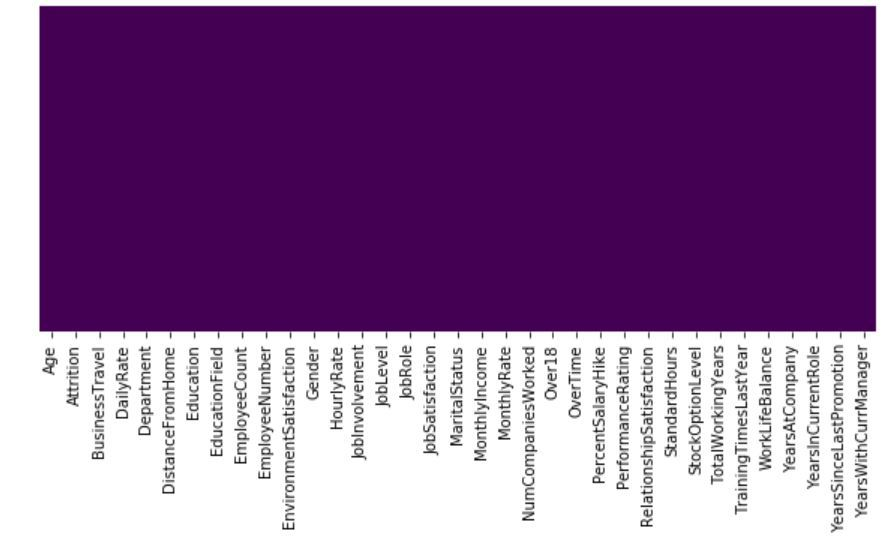So, we can see that there are no missing values in the dataset. This is a Binary Classification Problem, so the Distribution of instances among the 2 classes, is visualized below :

Code:

## Python3

 `sns.set_style(``'darkgrid'``)``sns.countplot(x ``=``'Attrition'``,``              ``data ``=` `dataset)`

Output: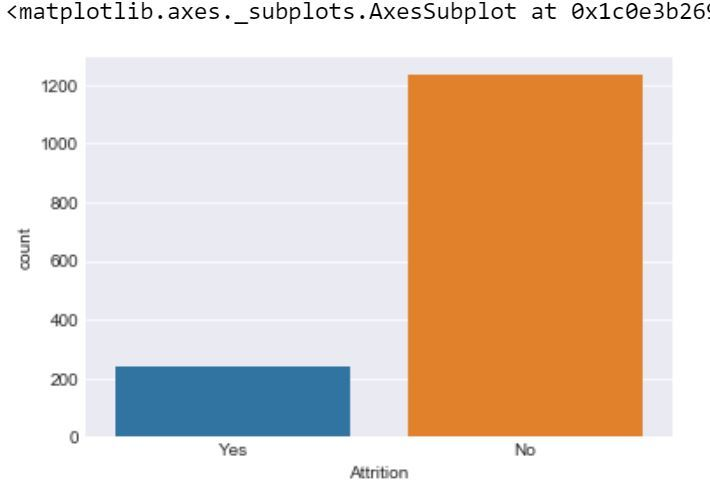Code:

## Python3

 `sns.lmplot(x ``=` `'Age'``,``           ``y ``=` `'DailyRate'``, ``           ``hue ``=` `'Attrition'``, ``           ``data ``=` `dataset)`

Output: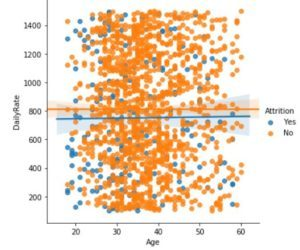Code:

## Python3

 `plt.figure(figsize ``=``(``10``, ``6``))``sns.boxplot(y ``=``'MonthlyIncome'``, ``            ``x ``=``'Attrition'``, ``            ``data ``=` `dataset)`

Output: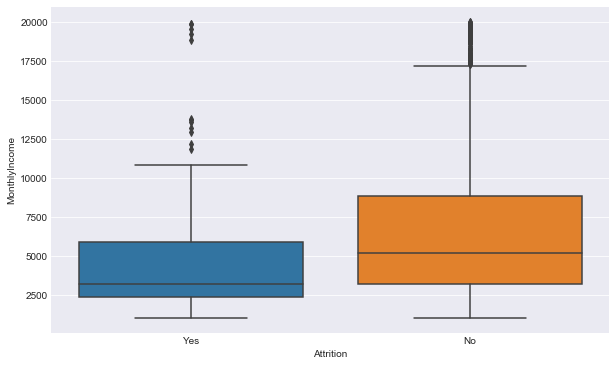Code: Preprocessing the data

In the dataset there are 4 irrelevant columns, i.e:EmployeeCount, EmployeeNumber, Over18, and StandardHour. So, we have to remove these for more accuracy.

## Python3

 `dataset.drop(``'EmployeeCount'``,``             ``axis ``=` `1``,``             ``inplace ``=` `True``)``dataset.drop(``'StandardHours'``,``             ``axis ``=` `1``,``             ``inplace ``=` `True``)``dataset.drop(``'EmployeeNumber'``,``             ``axis ``=` `1``, ``             ``inplace ``=` `True``)``dataset.drop(``'Over18'``, ``             ``axis ``=` `1``,``             ``inplace ``=` `True``)`` ` `print``(dataset.shape)`

Output:

```(1470, 31)
```

So, we have removed the irrelevant column.

Code: Input and Output data

## Python3

 `y ``=` `dataset.iloc[:, ``1``]``X ``=` `dataset`` ` `X.drop(``'Attrition'``,``       ``axis ``=` `1``, ``       ``inplace ``=` `True``)`

Code: Label Encoding

## Python3

 `from` `sklearn.preprocessing ``import` `LabelEncoder``lb ``=` `LabelEncoder()``y ``=` `lb.fit_transform(y)`

In the dataset there are 7 categorical data, so we have to change them to int data, i.e we have to create 7 dummy variables for better accuracy.

Code: Dummy variable creation

## Python3

 `dum_BusinessTravel ``=` `pd.get_dummies(dataset[``'BusinessTravel'``],``                                    ``prefix ``=``'BusinessTravel'``)`` ` `dum_Department ``=` `pd.get_dummies(dataset[``'Department'``],``                                ``prefix ``=``'Department'``)`` ` `dum_EducationField ``=` `pd.get_dummies(dataset[``'EducationField'``],``                                   ``prefix ``=``'EducationField'``)`` ` `dum_Gender ``=` `pd.get_dummies(dataset[``'Gender'``],``                           ``prefix ``=``'Gender'``, drop_first ``=` `True``)`` ` `dum_JobRole ``=` `pd.get_dummies(dataset[``'JobRole'``],`` ` `                            ``prefix ``=``'JobRole'``)`` ` `dum_MaritalStatus ``=` `pd.get_dummies(dataset[``'MaritalStatus'``],`` ` `                                  ``prefix ``=``'MaritalStatus'``)`` ` `dum_OverTime ``=` `pd.get_dummies(dataset[``'OverTime'``],``                             ``prefix ``=``'OverTime'``, drop_first ``=` `True``)`` ` `# Adding these dummy variable to input X``X ``=` `pd.concat([x, dum_BusinessTravel, dum_Department,``              ``dum_EducationField, dum_Gender, dum_JobRole,``              ``dum_MaritalStatus, dum_OverTime], axis ``=` `1``)`` ` `# Removing the categorical data``X.drop([``'BusinessTravel'``, ``'Department'``, ``'EducationField'``,``       ``'Gender'``, ``'JobRole'``, ``'MaritalStatus'``, ``'OverTime'``],``       ``axis ``=` `1``, inplace ``=` `True``)`` ` `print``(X.shape)``print``(y.shape)`

Output:

```(1470, 49)
(1470, )
```

Code: Splitting data to training and testing

## Python3

 `from` `sklearn.model_selection ``import` `train_test_split`` ` `X_train, X_test, y_train, y_test ``=` `train_test_split(`` ` `   ``X, y, test_size ``=` `0.25``, random_state ``=` `40``)`

So, the preprocessing is done, now we have to apply the Random forest classifier to the dataset.

Code: Model Execution

## Python3

 `from` `sklearn.model_selection ``import` `cross_val_predict, cross_val_score``from` `sklearn.metrics ``import` `accuracy_score, classification_report, confusion_matrix``from` `sklearn.ensemble ``import` `RandomForestClassifier`` ` `rf ``=` `RandomForestClassifier(n_estimators``=``10``,``                            ``criterion``=``'entropy'``)``rf.fit(X_train, y_train)``y_pred ``=` `rf.predict(X_test)`` ` `def` `print_score(clf, X_train, y_train,``                ``X_test, y_test,``                ``train``=``True``):`` ` `    ``if` `train:``        ``print``(``"Train Result:"``)``        ``print``(``"------------"``)``        ``print``(``"Classification Report: \n {}\n"``.``format``(classification_report(``            ``y_train, clf.predict(X_train))))`` ` `        ``print``(``"Confusion Matrix: \n {}\n"``.``format``(confusion_matrix(``            ``y_train, clf.predict(X_train))))`` ` `        ``res ``=` `cross_val_score(clf, X_train, y_train,``                              ``cv``=``10``, scoring``=``'accuracy'``)`` ` `        ``print``(``"Average Accuracy: \t {0:.4f}"``.``format``(np.mean(res)))``        ``print``(``"Accuracy SD: \t\t {0:.4f}"``.``format``(np.std(res)))``        ``print``(``"----------------------------------------------------------"``)`` ` `    ``elif` `train ``=``=` `False``:`` ` `        ``print``(``"Test Result:"``)``        ``print``(``"-----------"``)``        ``print``(``"Classification Report: \n {}\n"``.``format``(``            ``classification_report(y_test, clf.predict(X_test))))`` ` `        ``print``(``"Confusion Matrix: \n {}\n"``.``format``(``            ``confusion_matrix(y_test, clf.predict(X_test))))`` ` `        ``print``(``"accuracy score: {0:.4f}\n"``.``format``(``            ``accuracy_score(y_test, clf.predict(X_test))))`` ` `        ``print``(``"-----------------------------------------------------------"``)`` ` `print_score(rf, X_train, y_train,``            ``X_test, y_test,``            ``train``=``True``)`` ` `print_score(rf, X_train, y_train,``            ``X_test, y_test,``            ``train``=``False``)`

Output:

```Train Result:
------------
Classification Report:
precision    recall  f1-score   support

0       0.98      1.00      0.99       988
1       1.00      0.90      0.95       188

accuracy                           0.98      1176
macro avg       0.99      0.95      0.97      1176
weighted avg       0.98      0.98      0.98      1176

Confusion Matrix:
[[988   0]
[ 19 169]]

Average Accuracy:      0.8520
Accuracy SD:          0.0122
----------------------------------------------------------
Test Result:
-----------
Classification Report:
precision    recall  f1-score   support

0       0.86      0.98      0.92       245
1       0.71      0.20      0.32        49

accuracy                           0.85       294
macro avg       0.79      0.59      0.62       294
weighted avg       0.84      0.85      0.82       294

Confusion Matrix:
[[241   4]
[ 39  10]]

accuracy score: 0.8537

-----------------------------------------------------------
```

Code: Key features for deciding the result

## Python3

 `pd.Series(rf.feature_importances_, ``         ``index ``=` `X.columns).sort_values(ascending ``=` `False``).plot(kind ``=` `'bar'``, ``                                                                ``figsize ``=` `(``14``,``6``));`

Output: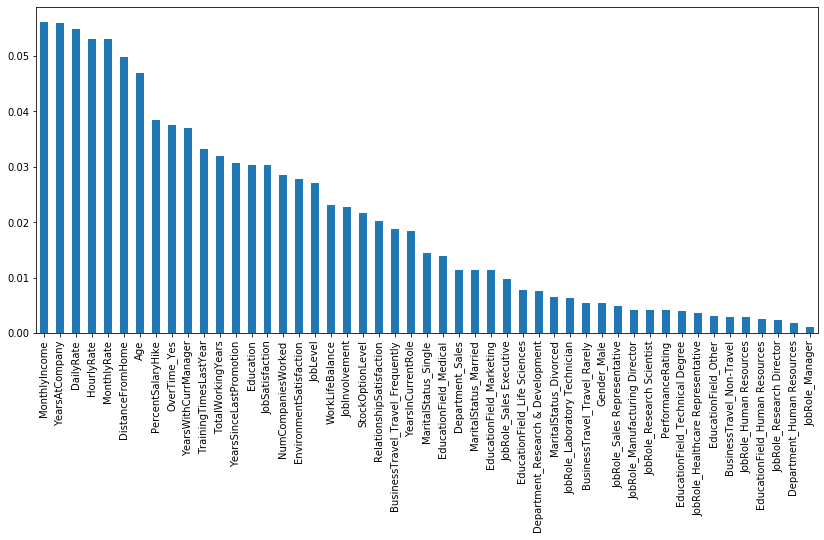So, According to Random forest classifier the most important feature for predicting the result is Monthly Income and the least important feature is jobRole_Manager.

My Personal Notes arrow_drop_up